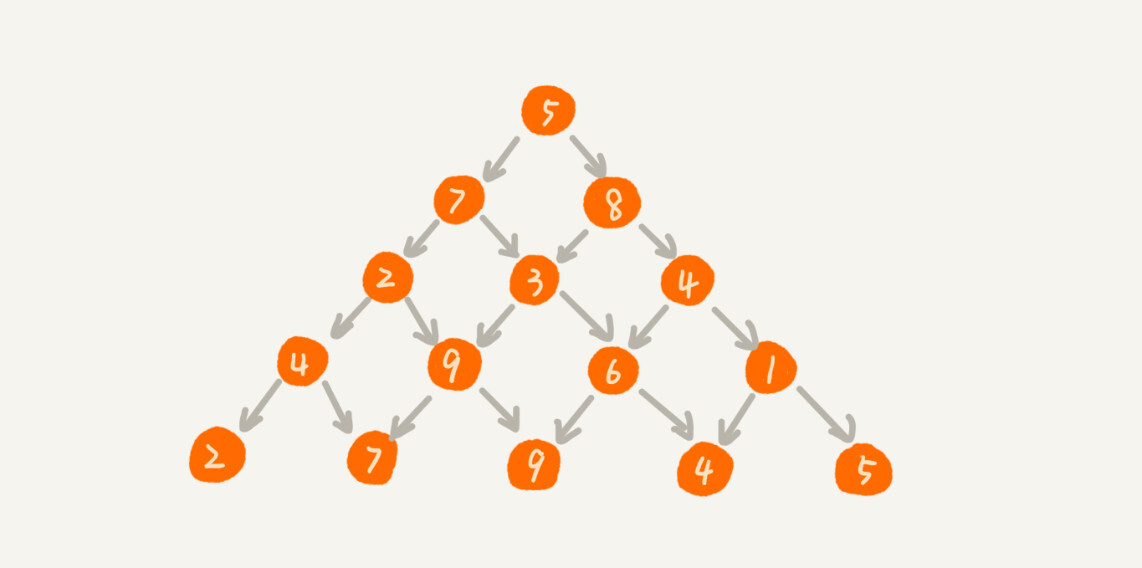## 0-1 背包问题

```// 回溯算法实现。注意：我把输入的变量都定义成了成员变量。
private int maxW = Integer.MIN_VALUE; // 结果放到maxW中
private int[] weight = {2，2，4，6，3};  // 物品重量
private int n = 5; // 物品个数
private int w = 9; // 背包承受的最大重量
public void f(int i, int cw) { // 调用f(0, 0)
if (cw == w || i == n) { // cw==w表示装满了，i==n表示物品都考察完了
if (cw > maxW) maxW = cw;
return;
}
f(i+1, cw); // 选择不装第i个物品
if (cw + weight[i] <= w) {
f(i+1,cw + weight[i]); // 选择装第i个物品
}
}```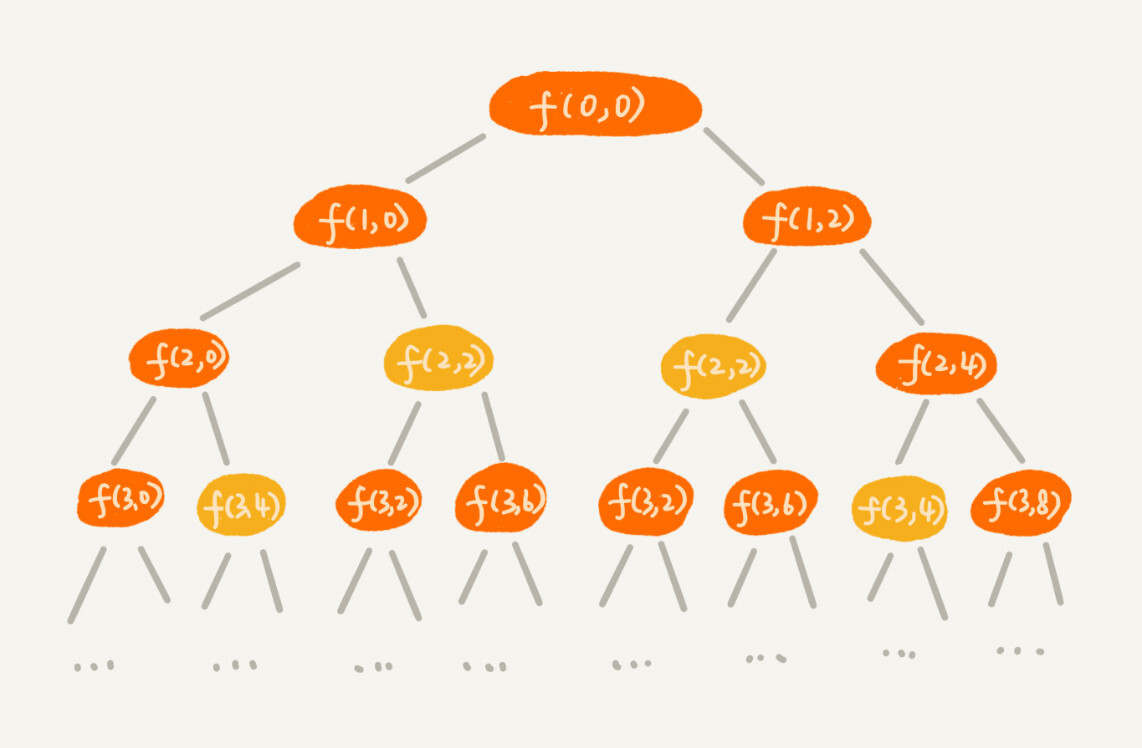```private int maxW = Integer.MIN_VALUE; // 结果放到maxW中
private int[] weight = {2，2，4，6，3};  // 物品重量
private int n = 5; // 物品个数
private int w = 9; // 背包承受的最大重量
private boolean[][] mem = new boolean; // 备忘录，默认值false
public void f(int i, int cw) { // 调用f(0, 0)
if (cw == w || i == n) { // cw==w表示装满了，i==n表示物品都考察完了
if (cw > maxW) maxW = cw;
return;
}
if (mem[i][cw]) return; // 重复状态
mem[i][cw] = true; // 记录(i, cw)这个状态
f(i+1, cw); // 选择不装第i个物品
if (cw + weight[i] <= w) {
f(i+1,cw + weight[i]); // 选择装第i个物品
}
}```

0 个（下标从 0 开始编号）物品的重量是 2，要么装入背包，要么不装入背包，决策完之后，会对应背包的两种状态，背包中物品的总重量是 0 或者 2。我们用 states=true 和 states=true 来表示这两种状态。

1 个物品的重量也是 2，基于之前的背包状态，在这个物品决策完之后，不同的状态有 3 个，背包中物品总重量分别是 0(0+0)，2(0+2 or 2+0)，4(2+2)。我们用 states=true，states=true，states=true 来表示这三种状态。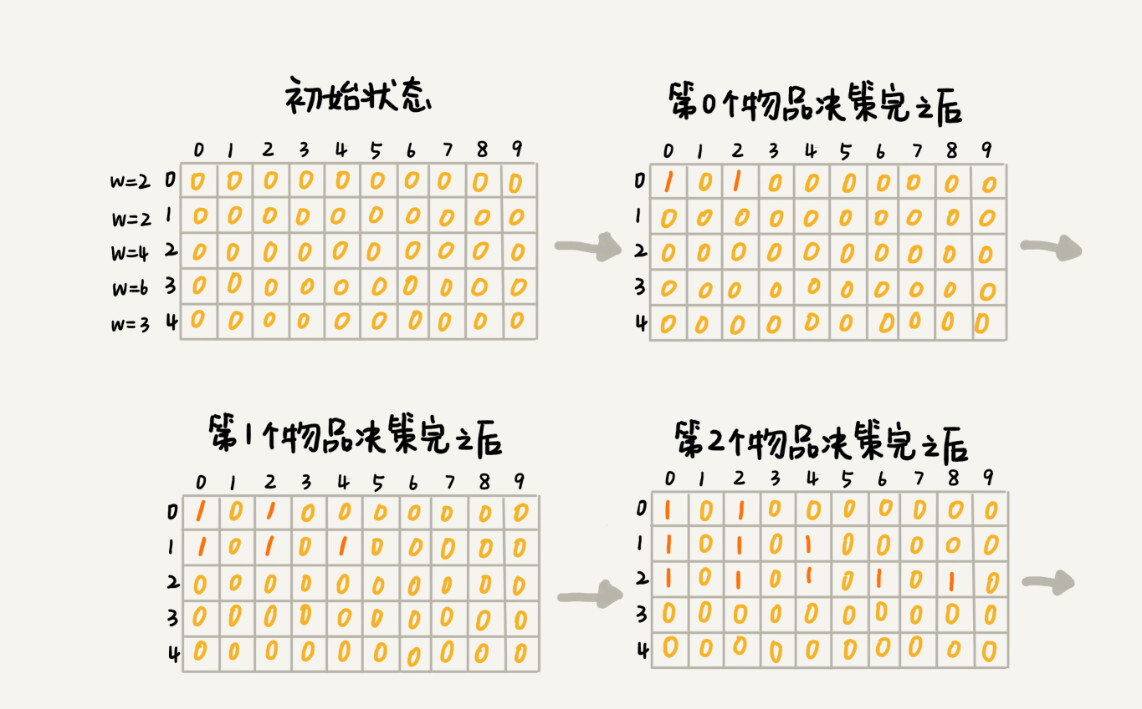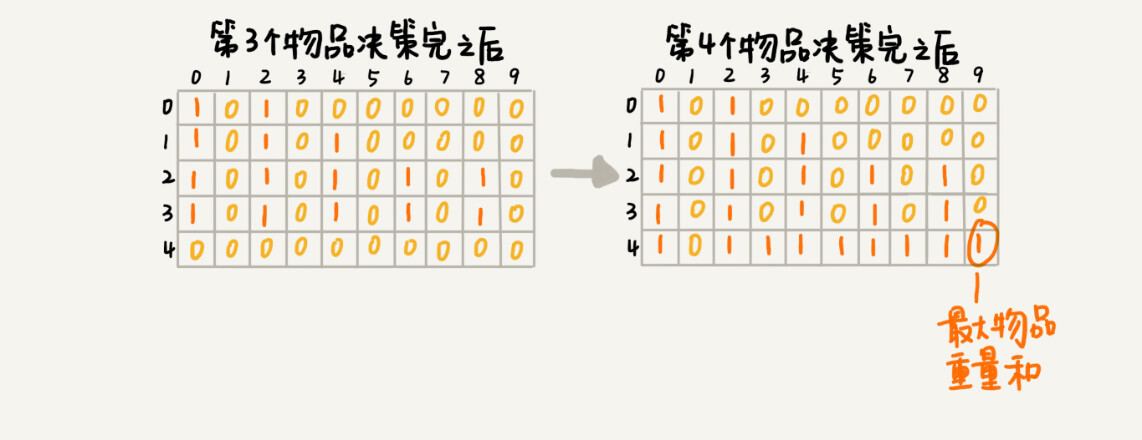```weight:物品重量，n:物品个数，w:背包可承载重量
public int knapsack(int[] weight, int n, int w) {
boolean[][] states = new boolean[n][w+1]; // 默认值false
states = true;  // 第一行的数据要特殊处理，可以利用哨兵优化
if (weight <= w) {
states[weight] = true;
}
for (int i = 1; i < n; ++i) { // 动态规划状态转移
for (int j = 0; j <= w; ++j) {// 不把第i个物品放入背包
if (states[i-1][j] == true) states[i][j] = states[i-1][j];
}
for (int j = 0; j <= w-weight[i]; ++j) {//把第i个物品放入背包
if (states[i-1][j]==true) states[i][j+weight[i]] = true;
}
}
for (int i = w; i >= 0; --i) { // 输出结果
if (states[n-1][i] == true) return i;
}
return 0;
}```

```public static int knapsack2(int[] items, int n, int w) {
boolean[] states = new boolean[w+1]; // 默认值false
states = true;  // 第一行的数据要特殊处理，可以利用哨兵优化
if (items <= w) {
states[items] = true;
}
for (int i = 1; i < n; ++i) { // 动态规划
for (int j = w-items[i]; j >= 0; --j) {//把第i个物品放入背包
if (states[j]==true) states[j+items[i]] = true;
}
}
for (int i = w; i >= 0; --i) { // 输出结果
if (states[i] == true) return i;
}
return 0;
}```

## 0-1 背包问题升级版

```private int maxV = Integer.MIN_VALUE; // 结果放到maxV中
private int[] items = {2，2，4，6，3};  // 物品的重量
private int[] value = {3，4，8，9，6}; // 物品的价值
private int n = 5; // 物品个数
private int w = 9; // 背包承受的最大重量
public void f(int i, int cw, int cv) { // 调用f(0, 0, 0)
if (cw == w || i == n) { // cw==w表示装满了，i==n表示物品都考察完了
if (cv > maxV) maxV = cv;
return;
}
f(i+1, cw, cv); // 选择不装第i个物品
if (cw + weight[i] <= w) {
f(i+1,cw+weight[i], cv+value[i]); // 选择装第i个物品
}
}```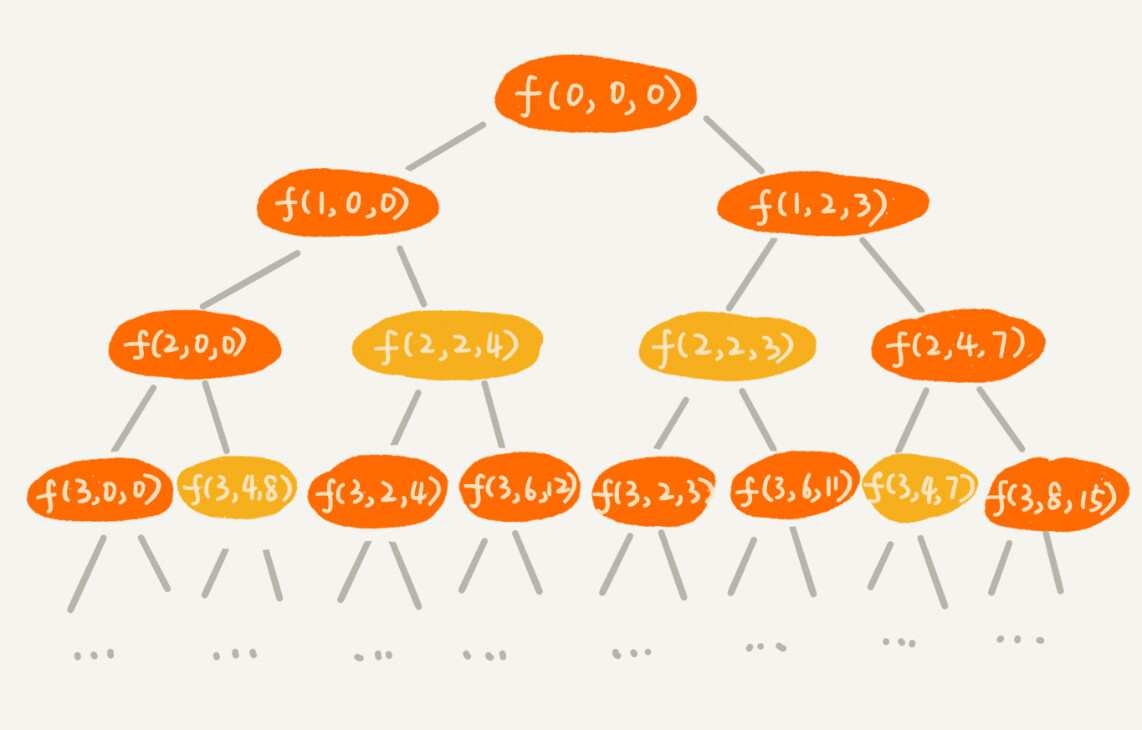```public static int knapsack3(int[] weight, int[] value, int n, int w) {
int[][] states = new int[n][w+1];
for (int i = 0; i < n; ++i) { // 初始化states
for (int j = 0; j < w+1; ++j) {
states[i][j] = -1;
}
}
states = 0;
if (weight <= w) {
states[weight] = value;
}
for (int i = 1; i < n; ++i) { //动态规划，状态转移
for (int j = 0; j <= w; ++j) { // 不选择第i个物品
if (states[i-1][j] >= 0) states[i][j] = states[i-1][j];
}
for (int j = 0; j <= w-weight[i]; ++j) { // 选择第i个物品
if (states[i-1][j] >= 0) {
int v = states[i-1][j] + value[i];
if (v > states[i][j+weight[i]]) {
states[i][j+weight[i]] = v;
}
}
}
}
// 找出最大值
int maxvalue = -1;
for (int j = 0; j <= w; ++j) {
if (states[n-1][j] > maxvalue) maxvalue = states[n-1][j];
}
return maxvalue;
}```

## 解答开篇

0-1 背包问题中，我们找的是小于等于 w 的最大值，x 就是背包的最大承载重量 w+1。对于这个问题来说，我们要找的是大于等于 200（满减条件）的值中最小的，所以就不能设置为 200 加 1 了。就这个实际的问题而言，如果要购买的物品的总价格超过 200 太多，比如 1000，那这个羊毛“薅”得就没有太大意义了。所以，我们可以限定 x 值为 1001。

```// items商品价格，n商品个数, w表示满减条件，比如200
public static void double11advance(int[] items, int n, int w) {
boolean[][] states = new boolean[n][3*w+1];//超过3倍就没有薅羊毛的价值了
states = true;  // 第一行的数据要特殊处理
if (items <= 3*w) {
states[items] = true;
}
for (int i = 1; i < n; ++i) { // 动态规划
for (int j = 0; j <= 3*w; ++j) {// 不购买第i个商品
if (states[i-1][j] == true) states[i][j] = states[i-1][j];
}
for (int j = 0; j <= 3*w-items[i]; ++j) {//购买第i个商品
if (states[i-1][j]==true) states[i][j+items[i]] = true;
}
}

int j;
for (j = w; j < 3*w+1; ++j) {
if (states[n-1][j] == true) break; // 输出结果大于等于w的最小值
}
if (j == 3*w+1) return; // 没有可行解
for (int i = n-1; i >= 1; --i) { // i表示二维数组中的行，j表示列
if(j-items[i] >= 0 && states[i-1][j-items[i]] == true) {
System.out.print(items[i] + " "); // 购买这个商品
j = j - items[i];
} // else 没有购买这个商品，j不变。
}
if (j != 0) System.out.print(items);
}```

## 课后思考

“杨辉三角”不知道你听说过吗？我们现在对它进行一些改造。每个位置的数字可以随意填写，经过某个数字只能到达下面一层相邻的两个数字。